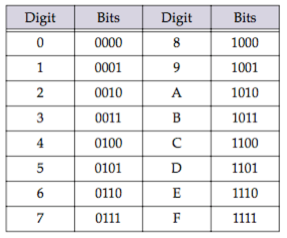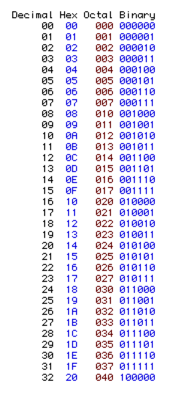The number of digits a numeric system uses is called its radix. The radix of a number is often displayed as a subscript at the end of the number. For example 101 base 2 (binary) is the same value as 5 base 10 (decimal). If you’ve never been exposed to non-decimal radix numbers before, this whole concept probably seems quite strange. However, for raw binary information, non-decimal numbers (especially hex) are much easier to work with, and they are universally used by programmers from the largest mainframe to the smallest microcomputer.

For raw data, programmers use numbers that are base 2 (binary), base 8 (octal), or base 16 (hexadecimal or just “hex”). All these number systems are convenient for working with raw data because they are powers of two. When using base 2, there are 2 digits (0 and 1) and each digit represents a power of 2. When using base 8, there are 8 digits (0, 1, 2, 3, 4, 5, 6, 7) and each digit represents a power of 8. When using base 16 there are 16 digits (0, 1, 2, 3, 4, 5, 6, 7, 8, 9, A, B, C, D, E, F) and each digit represents a power of 16. Hex numbers are most programmers favorite because each digit corresponds to four bits:The table below shows the equivalent decimal, hex, octal and binary numbers from 0 to 32 (decimal).